# 1.6. 最近邻

sklearn.neighbors 提供了 neighbors-based (基于邻居的) 无监督学习以及监督学习方法的功能。 无监督的最近邻是许多其它学习方法的基础，尤其是 manifold learning (流形学习) 和 spectral clustering (谱聚类)。 neighbors-based (基于邻居的) 监督学习分为两种： classification （分类）针对的是具有离散标签的数据，regression （回归）针对的是具有连续标签的数据。

sklearn.neighbors 可以处理 Numpy 数组或 scipy.sparse 矩阵作为其输入。 对于密集矩阵，大多数可能的距离度量都是支持的。对于稀疏矩阵，支持搜索任意的 Minkowski 度量。

## 1.6.1. 无监督最近邻

NearestNeighbors （最近邻）实现了 unsupervised nearest neighbors learning（无监督的最近邻学习）。 它为三种不同的最近邻算法提供统一的接口：BallTree, KDTree, 还有基于 sklearn.metrics.pairwise 的 brute-force 算法。算法的选择可通过关键字 'algorithm' 来控制， 并必须是 ['auto', 'ball_tree', 'kd_tree', 'brute'] 其中的一个。当设置为默认值 'auto' 时，算法会尝试从训练数据中确定最佳方法。有关上述每个选项的优缺点，参见 Nearest Neighbor Algorithms_

• 关于最近邻算法，如果邻居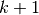和邻居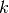具有相同的距离，但具有不同的标签， 结果将取决于训练数据的顺序。

### 1.6.1.1. 找到最近邻

>>> from sklearn.neighbors import NearestNeighbors
>>> import numpy as np
>>> X = np.array([[-1, -1], [-2, -1], [-3, -2], [1, 1], [2, 1], [3, 2]])
>>> nbrs = NearestNeighbors(n_neighbors=2, algorithm='ball_tree').fit(X)
>>> distances, indices = nbrs.kneighbors(X)
>>> indices
array([[0, 1],
[1, 0],
[2, 1],
[3, 4],
[4, 3],
[5, 4]]...)
>>> distances
array([[0.        , 1.        ],
[0.        , 1.        ],
[0.        , 1.41421356],
[0.        , 1.        ],
[0.        , 1.        ],
[0.        , 1.41421356]])


>>> nbrs.kneighbors_graph(X).toarray()
array([[ 1.,  1.,  0.,  0.,  0.,  0.],
[ 1.,  1.,  0.,  0.,  0.,  0.],
[ 0.,  1.,  1.,  0.,  0.,  0.],
[ 0.,  0.,  0.,  1.,  1.,  0.],
[ 0.,  0.,  0.,  1.,  1.,  0.],
[ 0.,  0.,  0.,  0.,  1.,  1.]])


### 1.6.1.2. KDTree 和 BallTree 类

>>> from sklearn.neighbors import KDTree
>>> import numpy as np
>>> X = np.array([[-1, -1], [-2, -1], [-3, -2], [1, 1], [2, 1], [3, 2]])
>>> kdt = KDTree(X, leaf_size=30, metric='euclidean')
>>> kdt.query(X, k=2, return_distance=False)
array([[0, 1],
[1, 0],
[2, 1],
[3, 4],
[4, 3],
[5, 4]]...)


## 1.6.2. 最近邻分类

scikit-learn 实现了两种不同的最近邻分类器： 基于每个查询点的个最近邻实现，其中是用户指定的整数值。RadiusNeighborsClassifier 基于每个查询点的固定半径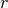内的邻居数量实现， 其中是用户指定的浮点数值。-邻居分类是KNeighborsClassifie的技术中比较常用的一种。 值的最佳选择是高度依赖数据的：通常较大的是会抑制噪声的影响，但是使得分类界限不明显。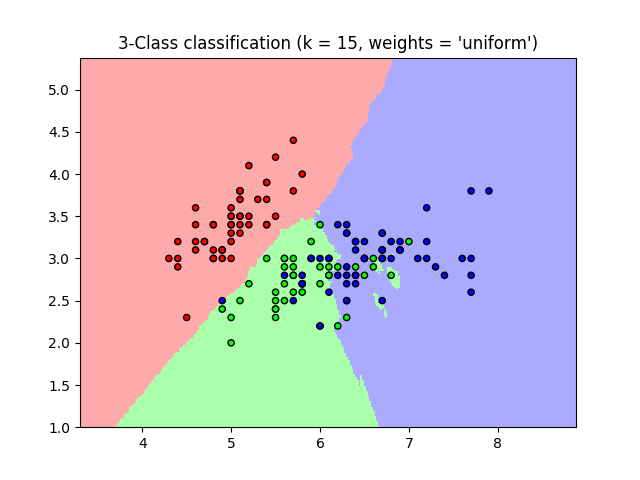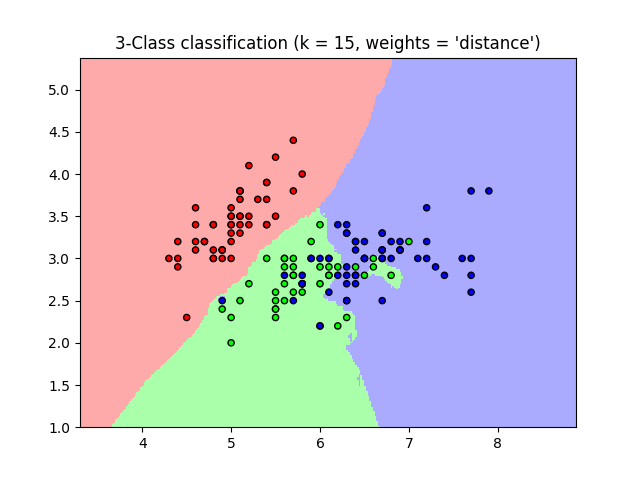## 1.6.3. 最近邻回归

scikit-learn 实现了两种不同的最近邻回归：KNeighborsRegressor 基于每个查询点的个最近邻实现， 其中是用户指定的整数值。RadiusNeighborsRegressor 基于每个查询点的固定半径内的邻点数量实现， 其中是用户指定的浮点数值。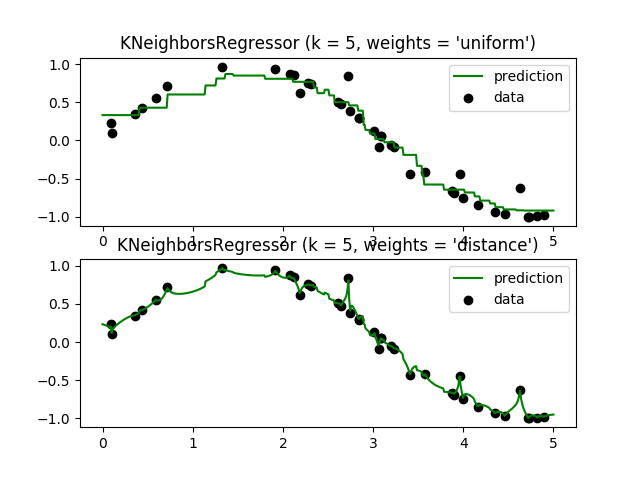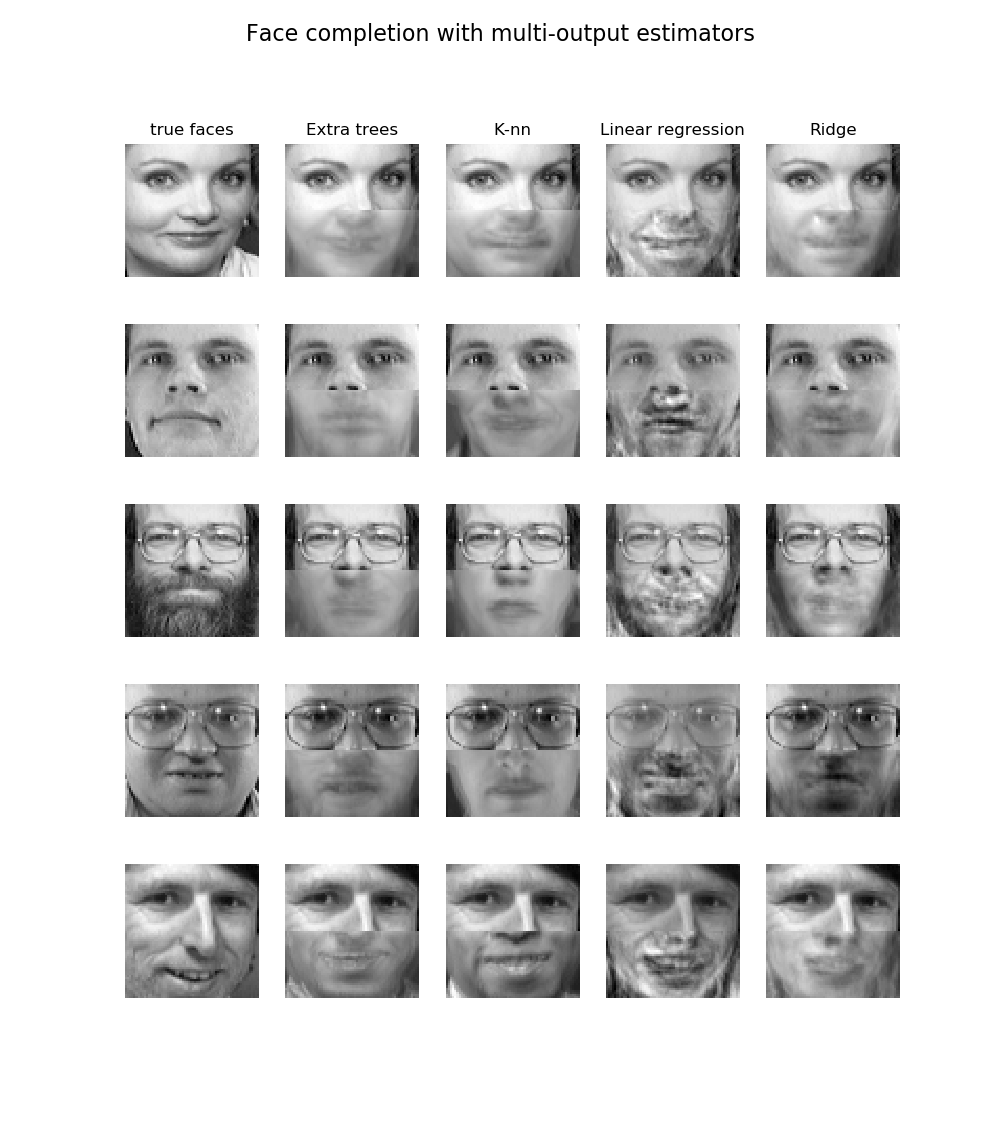## 1.6.4. 最近邻算法

### 1.6.4.3. Ball 树

ball 树将数据递归地划分为由质心 C 和半径 R 定义的节点,使得节点中的每个点位于由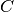定义的 hyper-sphere 内. 通过使用 triangle inequality（三角不等式） 减少近邻搜索的候选点数: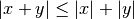### 1.6.4.4. 最近邻算法的选择

• 样本数量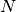(i.e. n_samples) 和维度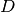(例如. nfeatures).

• Brute force 查询时间以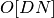增长

• Ball tree 查询时间大约以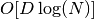增长

• KD tree 的查询时间 的变化是很难精确描述的.对于较小的(小于20) 的成本大约是, 并且 KD 树更加有效.对于较大的成本的增加接近, 由于树结构引起的开销会导致查询效率比暴力还要低.

对于小数据集 (n小于30), log(N)相当于N, 暴力算法比基于树的算法更加有效. KDTreeBallTree 通过提供一个 leaf size 参数来解决这个问题: 这控制了查询切换到暴力计算样本数量. 使得两种算法对较小的的效率都能接近于暴力计算.

• 数据结构: 数据的 intrinsic dimensionality (本征维数) 和/或数据的 sparsity (稀疏度). 本征维数是指数据所在的流形的维数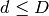, 在参数空间可以是线性或非线性的. 稀疏度指的是数据填充参数空间的程度(这与“稀疏”矩阵中使用的概念不同, 数据矩阵可能没有零项, 但是从这个意义上来讲,它的 structure 仍然是 “稀疏” 的)。

• Brute force (暴力查询)时间不受数据结构的影响。

• Ball treeKD tree 的数据结构对查询时间影响很大. 一般地, 小维度的 sparser (稀疏) 数据会使查询更快. 因为 KD 树的内部表现形式是与参数轴对齐的, 对于任意的结构化数据它通常不会表现的像 ball tree 那样好.

在机器学习中往往使用的数据集是非常结构化的, 而且非常适合基于树结构的查询。

• query point（查询点）所需的近邻数> Brute force 查询时间几乎不受值的影响. > Ball treeKD tree 的查询时间会随着的增加而变慢. 这是由于两个影响: 首先,的值越大在参数空间中搜索的部分就越大. 其次, 使用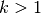进行树的遍历时, 需要对内部结果进行排序.相比变大时, 在基于树的查询中修剪树枝的能力是减弱的. 在这种情况下, 暴力查询会更加有效.

• query points（查询点）数. ball tree 和 KD Tree 都需要一个构建阶段. 在许多查询中分摊时，这种结构的成本可以忽略不计。 如果只执行少量的查询, 可是构建成本却占总成本的很大一部分. 如果仅需查询很少的点, 暴力方法会比基于树的方法更好.

### 1.6.4.5. leaf_size 的影响

leaf_size 不被 brute force queries（暴力查询）所引用.

## 1.6.5. 最近质心分类

NearestCentroid 分类器是一个简单的算法, 通过其成员的质心来表示每个类。 实际上, 这使得它类似于 sklearn.KMeans 算法的标签更新阶段. 它也没有参数选择, 使其成为良好的基准分类器. 然而，它确实受到非凸类的影响，即当类有显著不同的方差时。所以这个分类器假设所有维度的方差都是相等的。 对于没有做出这个假设的更复杂的方法, 请参阅线性判别分析 (sklearn.discriminant_analysis.LinearDiscriminantAnalysis) 和二次判别分析 (sklearn.discriminant_analysis.QuadraticDiscriminantAnalysis). 默认的 NearestCentroid 用法示例如下:

>>> from sklearn.neighbors.nearest_centroid import NearestCentroid
>>> import numpy as np
>>> X = np.array([[-1, -1], [-2, -1], [-3, -2], [1, 1], [2, 1], [3, 2]])
>>> y = np.array([1, 1, 1, 2, 2, 2])
>>> clf = NearestCentroid()
>>> clf.fit(X, y)
NearestCentroid(metric='euclidean', shrink_threshold=None)
>>> print(clf.predict([[-0.8, -1]]))




### 1.6.5.1. 最近缩小质心

NearestCentroid 分类器有一个 shrink_threshold 参数, 它实现了 nearest shrunken centroid 分类器. 实际上, 每个质心的每个特征的值除以该特征的类中的方差. 然后通过 shrink_threshold 来减小特征值. 最值得注意的是, 如果特定特征值过0, 则将其设置为0. 实际上，这个方法移除了影响分类器的特征。 这很有用, 例如, 去除噪声特征.

## 1.6.6 邻域成分分析

#### 1.6.6.1. 分类

NCA分类在不同规模和难度的数据集的实际应用中显示出良好的效果。与线性判别分析等相关方法相比，NCA没有对类的分布做任何假设。而最近邻分类自然会产生高度不规则的决策边界。

>>> from sklearn.neighbors import (NeighborhoodComponentsAnalysis,
... KNeighborsClassifier)
>>> from sklearn.model_selection import train_test_split
>>> from sklearn.pipeline import Pipeline
>>> X_train, X_test, y_train, y_test = train_test_split(X, y,
... stratify=y, test_size=0.7, random_state=42)
>>> nca = NeighborhoodComponentsAnalysis(random_state=42)
>>> knn = KNeighborsClassifier(n_neighbors=3)
>>> nca_pipe = Pipeline([('nca', nca), ('knn', knn)])
>>> nca_pipe.fit(X_train, y_train)
Pipeline(...)
>>> print(nca_pipe.score(X_test, y_test))
0.96190476...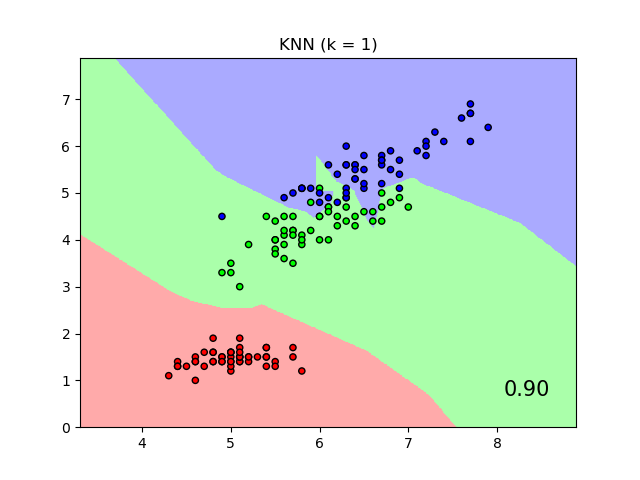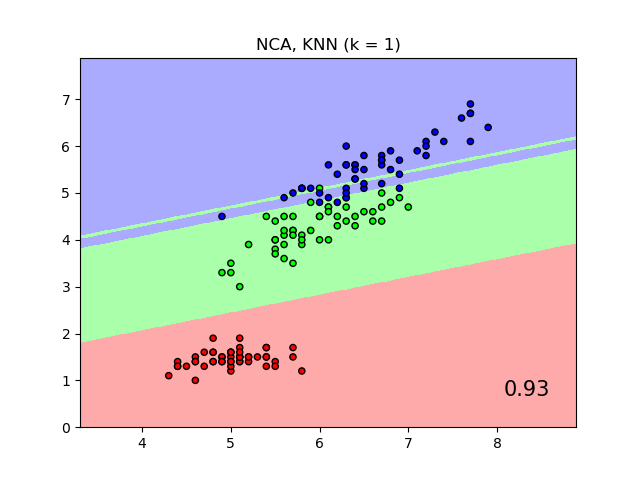#### 1.6.6.2. 降维

NCA可用于进行监督降维。输入数据被投影到一个由最小化NCA目标的方向组成的线性子空间上。可以使用参数n_components设置所需的维数。例如,下图显示了主成分分析的降维(sklearn.decomposition.PCA),线性判别分析(sklearn.discriminant_analysis.LinearDiscriminantAnalysis)和邻域成分分析(NeighborhoodComponentsAnalysis)在一个64个特征,1797个样本的数字数据集的降维结果。数据集被划分为大小相同的训练集和测试集，然后进行标准化。为了评价该方法的分类精度，对每种方法找到的二维投影点进行了3-最近邻分类精度的计算。每个数据样本属于10个类中的一个。

#### 1.6.6.3 数学公式

NCA的目标是拟合一个尺寸为(n_components, n_features)的最优线性变换矩阵，使所有被正确分类的概率样本的和最大，即: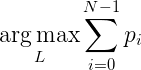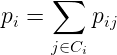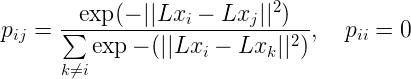##### 1.6.6.3.1 Mahalanobis距离

NCA可以看作是拟合一个(平方)Mahalanobis距离矩阵: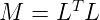是大小为(特征数，特征数)对称正半定矩阵．

#### 1.6.6.5 复杂度

##### 1.6.6.5.1 训练

NCA存储一对距离矩阵，占用了（n_samples ** 2）的内存。时间复杂度取决于优化算法的迭代次数。但是，可以使用参数｀max_iter｀设置迭代的最大次数。对于每个迭代，时间复杂度为O(n_components x n_samples x min(n_samples, n_features))